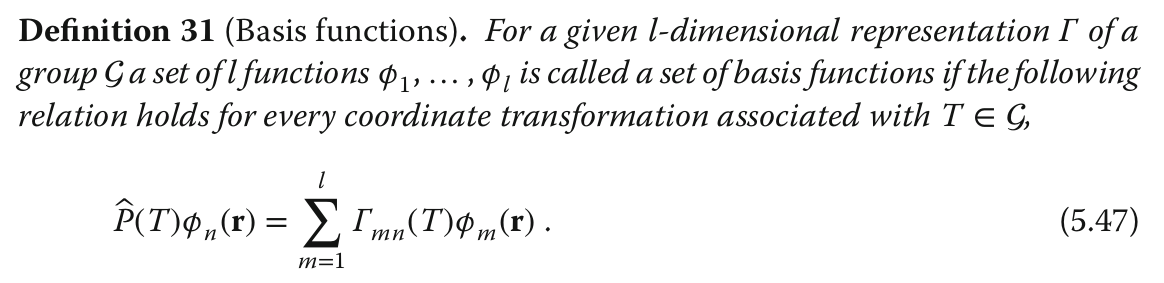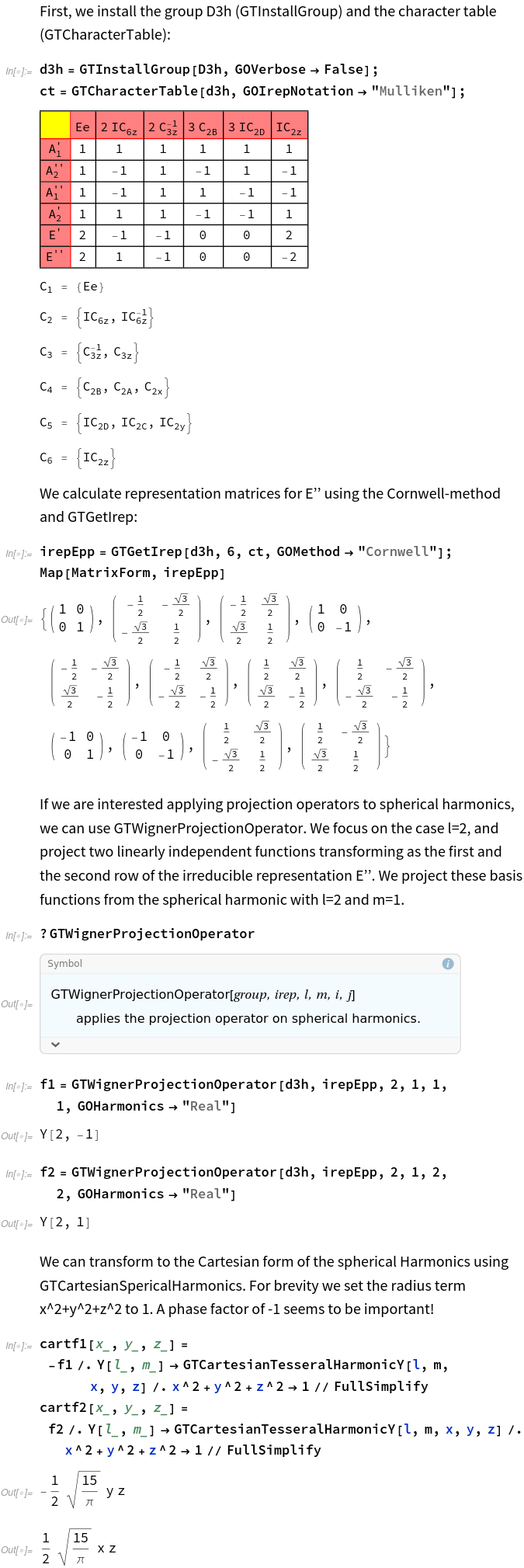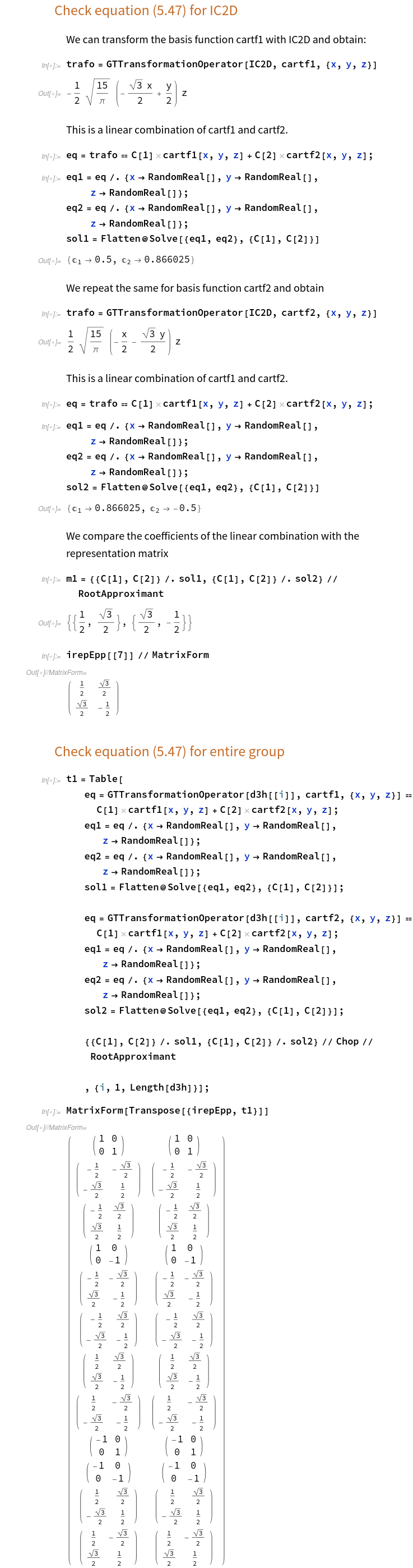# How to get the basis functions for a given matrix realization of an irrep?

398 views

Hello,

As far as I understand, once GTPack provides a matrix realization for an irrep of a point group (through any method: Cornwell, Flodmark, Induction), one should be able to obtain symmetry-adapted linear combinations of e.g. spherical harmonics that transform exactly according to that matrix realization, i.e. that verify Eq. (5.47) of the GTPack book. Although I intend ultimately to treat rather general cases, I am having problems at present with a simple case, namely the two-dimensional irrep E” of group D3h. By using the command GTWignerProjectionOperator I arrive to the conclusion that the basis functions of the matrix irrep (obtained by Cornwell method) should be:

phi1 = 1/2 Y[2,-1]+1/2 Y[2,1] –> y z
phi2 = 1/2 Y[2,-1]-1/2 Y[2,1] –> x z

BUT the problem is that, when I try to check that these functions verify Eq. (5.47) they don’t !!! (at least not for all symmetry operations)

I repeat the whole procedure for irrep matrices obtained by Flodmark and Induction methods and get the same (if not worst) result.

(I like to think the symmetry operations as active so I am using GTReinstallAxes[“active”]. I do not know if this is of any relevance).

Can anybody help me?

Alberto.

Hi Alberto,

Thanks for the great question! I realized an inconsistency combining the Cornwell method and GTWignerProjectionOperator. The Cornwell method uses real spherical Harmonics, while GTWignerProjectionOperator used complex spherical harmonics so far. I uploaded a quick bug fix (GTPack 1.32) which allows for real spherical harmonics in GTWignerProjectionOperator.

For general understanding, I add the definition around equation (5.47) of our book here. It concerns the transformation behavior of basis functions under the symmetry elements of a group:I also prepared a notebook which compares the projected basis functions with the representation obtained by the Cornwell method. However, in the projection scheme there is an ambiguous phase. Hence, in general, matrices we reconstruct are similar, but not necessarily identical. You can either find the global similarity transformation between initial and reconstructed representation, or try to find the correct phase at the beginning.

Let me know if the code below helps to answer your question.

-Matthias